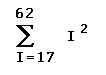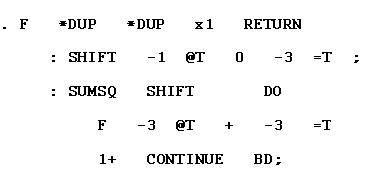Previous Chapter

An Example

We illustrate the language of FORTH by summing the squares of integers between 2 limits. The user types

``` 17 63 SUMSQ```

To requestFORTH source language to evaluate and type a sum of squares with limits on the stack.

Fig. 8 shows the source language required. We will attempt a running commentary:

Since the numbers 17 and 63 are not in the dictionary, the scanner places them on the stack. It then find SUMSQ in the dictionary and executes the associated code. The colon preceding SUMSQ indicates that it is a definition, so the scanner is directed to the character string following it. The next word read is thus SHIFT.

SHIFT must also be interpreted. At this point the stack contains 17 63. It will place a zero before the numbers on the stack (interchanging them in the process).

-1 @T are 2 words that form a phrase. -1 is a number that is placed on the stack. @T uses it as an address relative to the top of the stack and fetches the first number - 17. 0 is a number and is placed on the stack. -3 =T is another phrase that will store this 0 3 places deep in the stack - where the 17 was. At this point the stack contains 0 63 17.

The ; marking the end of SHIFT directs the scanner back to SUMSQ where the next word is DO. DO marks the beginning of a loop.

Next Chapter